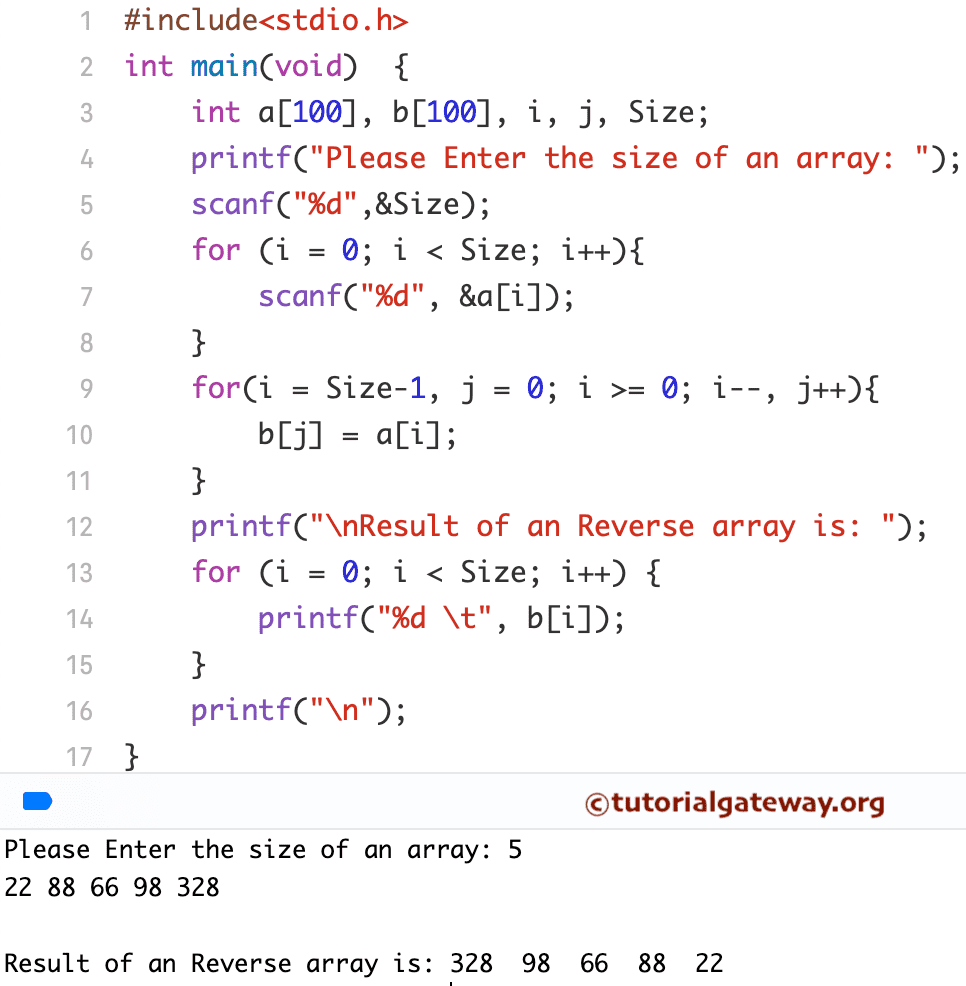# What is the logic to reverse the array?

What is the logic to reverse the array?

• 4 Answer(s)

# C program to reverse an array:

C program to reverse an array: This program reverses the array elements. For example, if ‘A’ is an array of integers with three elements such that
A = 1, A = 2, A = 3
Then after reversing, the array will be
A = 3, A = 2, A = 1

## Reverse array C program

1. #include <stdio.h>
2. int main()
3. {
4.    int n, c, d, a, b;
5.    printf(“Enter the number of elements in array\n”);
6.    scanf(“%d”, &n);
7.    printf(“Enter array elements\n”);
8.    for (c = 0; c < n ; c++)
9.       scanf(“%d”, &a[c]);
10.    /*
11.     * Copying elements into array b starting from end of array a
12.     */
13.    for (c = n – 1, d = 0; c >= 0; c–, d++)
14.       b[d] = a[c];
15.    /*
16.     * Copying reversed array into the original.
17.     * Here we are modifying original array, this is optional.
18.     */
19.    for (c = 0; c < n; c++)
20.       a[c] = b[c];
21.    printf(“Reverse array is\n”);
22.    for (c = 0; c < n; c++)
23.       printf(“%d\n”, a[c]);
24.    return 0;
25. }

Reverse array C program output:Logic to find reverse of array
The above program prints array in reversed order. It does not reverses array. Here I am writing first basic logic to reverse array. It uses above approach to access array element in reverse and copy it to a new reverse array. Which means last element of original array becomes the first element for reverse array.

Step by step descriptive logic to reverse an array.

Input size and elements in an array. Store it in some variable say size and arr respectively.
Declare another array that will store reversed array elements of original array with same size, say reverse[size].
Initialize two variables that will keep track of original and reverse array. Here we will access original array from last and reverse array from first. Hence, initialize arrIndex = size – 1 and revIndex = 0.
Run loop from size – 1 to 0 in decremented style. The loop structure should look like while(arrIndex >= 0).
Inside loop copy original array to reverse array i.e. reverse [revIndex] = arr[arrIndex];.
After copy, increment revIndex and decrement arrIndex.
Finally after loop print reverse array.
Program to find reverse of array
/**
* C program to find reverse of array
*/

#include
#define MAX_SIZE 100 // Maximum array size

int main()
{
int arr[MAX_SIZE], reverse[MAX_SIZE];
int size, i, arrIndex, revIndex;

/* Input size of the array */
printf(“Enter size of the array: “);
scanf(“%d”, &size);

/* Input array elements */
printf(“Enter elements in array: “);
for(i=0; i= 0)
{
/* Copy value from original array to reverse array */
reverse[revIndex] = arr[arrIndex];

revIndex++;
arrIndex–;
}

/*
* Print the reversed array
*/
printf(“\nReversed array : “);
for(i=0; i

## C Program to Reverse an Array

``

C program to reverse an array: This program reverses the array elements. For example, if ‘A’ is an array of integers with three elements such that
A = 1, A = 2, A = 3
Then after reversing, the array will be
A = 3, A = 2, A = 1

• ### Your Answer

By posting your answer, you agree to the privacy policy and terms of service.